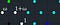# My journey with unit testing in Java so farThese are three of the units in the ring of Eisenstein integers (white dots with white labels). A little off-topic, at least at the beginning.
`    @Test    public void fakeTest() {        System.out.println("Fake test");    }`
`int expResult = 2;int result = 1 + 1;assertEquals(expResult, result);`
`GaussianInteger expResult = new GaussianInteger(-1, 0);GaussianInteger imagUnit = new GaussianInteger(0, 1);GaussianInteger result = imagUnit.times(imagUnit);assertEquals(expResult, result);`
`@Test(expected = Exception.class)public void testDividingByZero() {    System.out.println("Testing division by zero.");    QuadraticRing OQi7 = new QuadraticRing(-7);    QuadraticInteger dividend = new QuadraticInteger(-3, 2, OQi7);    QuadraticInteger divisor = new QuadraticInteger(0, 0, OQi7);    QuadraticInteger result = dividend.dividedBy(divisor);}`
`    dividend = new QuadraticInteger(-3, 2, OQi7);    divisor = new QuadraticInteger(0, 0, OQi7);    try {        result = dividend.dividedBy(divisor);        fail("Division by 0 did not trigger any exception.");    } catch (ArithmeticException ae) {        System.out.println("Division by 0 correctly triggered ArithmeticException.");    } catch (IllegalArgumentException iae) {        System.out.println("Division by 0 correctly triggered IllegalArgumentException.");    } catch (Exception e) {        fail("Division by 0 triggered the wrong exception," + e.getMessage());    }`
`public class NumberTheoreticFunctionsCalculator {`
`import org.junit.After;import org.junit.AfterClass;import org.junit.Before;import org.junit.BeforeClass;import org.junit.Test;import static org.junit.Assert.*;`
`    fail("The test case is a prototype.");`
`  ImaginaryQuadraticInteger gaussInt = new ImaginaryQuadraticInteger(a, b, ringGaussian, 1);`
`  ImaginaryQuadraticInteger gaussInt = new ImaginaryQuadraticInteger(a, b, ringGaussian);`

is a composer and photographer from Detroit, Michigan. He has been working on a Java program to display certain mathematical diagrams.

## More from Alonso Del Arte

is a composer and photographer from Detroit, Michigan. He has been working on a Java program to display certain mathematical diagrams.